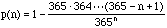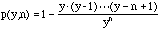Date: Fri, 16 Apr 1999 11:52:13 -0600 (CST)
Sender: Gordon
Subject: the birthday problem

Who is asking: Teacher
Level: Secondary

Question:
How do I explain the rapid rise in the probability that at least two people in a group of n have the same birthday. We have derived the formula for p(n) and have graphed it and have seen how the results are counter-intuitive. At around n=23 p(n)=.5 and at n=50 p(n) is very close to 1. It does not help to simplify the problem (eg use months instead of days) because then our intuition does correspond more closely to reality. Is there some way we can see how the probabiltiy of a "collision" increases with n? It makes me think of data storage problems and hash tables in computer science. thanks if you can help

Hi Gordon

This is a difficult problem. I am not sure that there is a way to explain the birthday problem that is intuitive. The only way that I see it is from the form of the expressionThe key here is that in the fractional part both the numerator and denominator increase with increasing n, but the denominator increases at a much faster rate than the numerator.
Your comment that simplifying the problem by using months (I think of this as a 12 day year) where n = 5 gives p(n) approxiamtely 0.5, made me wonder what happens for "years" of different lengths. If a year is y days long then the appropriate probability, now a function of y and n isThe birthday problem is then, for each y, find the smallest n that gives p(y,n) > 0.5. If this number is called f(y) then the traditional birthday problem has answer f(365) = 23 and your "12 day year" problem gives f(12) = 5. In fact f(y) = 5 if y is anywhere from 10 to 16 days. I did some calculations and produced the following table

f(y)Range of y
510 to 16
617 to 23
724 to 32
833 to 42
943 to 54
1055 to 68
1169 to 82
1283 to 99
13100 to 116
14117 to 135
15136 to 156
16157 to 178
17179 to 201
18202 to 226
19227 to 252
20253 to 280
21281 to 309
22310 to 340
23341 to 372

This is not an answer to your question but experimenting with the numbers does help me understand why n = 23 is the correct answer to the birthday problem even though it is still counter-intuitive.

Cheers
Harley

Go to Math Central

To return to the previous page use your browser's back button.